Hi,
(1) The Introduction to simulation indicate that the ref_pos is generated by true GPS.
Q: Which measurement method of GPS is used here? Differential GPS or Real Time Kinematic?
(2) When I use the INS algorithm to do simulation, the reference trajectory and algorithm trajectory are very different. Is there a problem with my settings?
The screenshots of my model sensor, motion and run are：The motion generator used is the Drive case comes with system.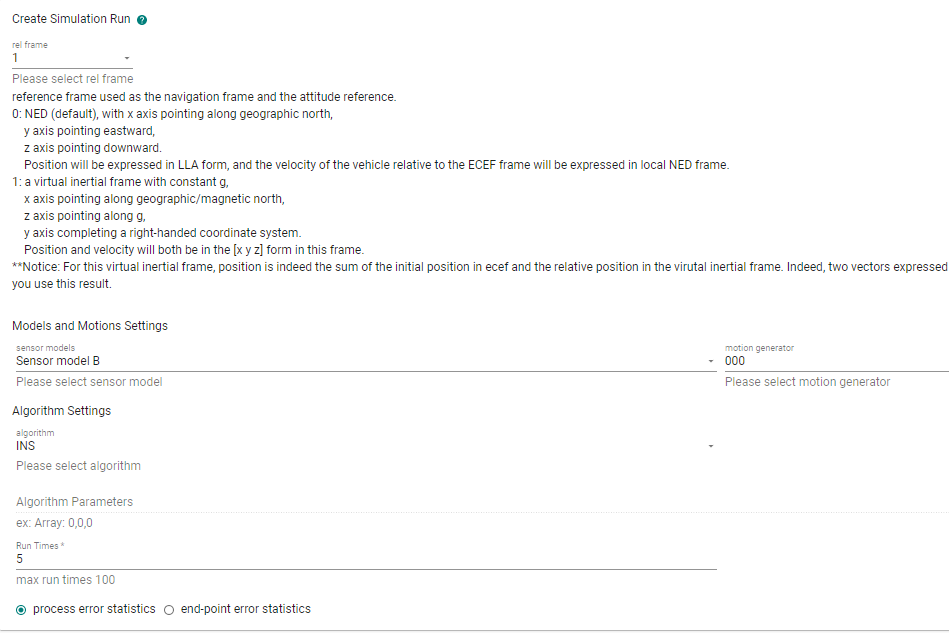Here are the screenshots of pos_algo_0 compare with ref_pos( ref_pos is the green line, pos_algo_0 is the yellow line):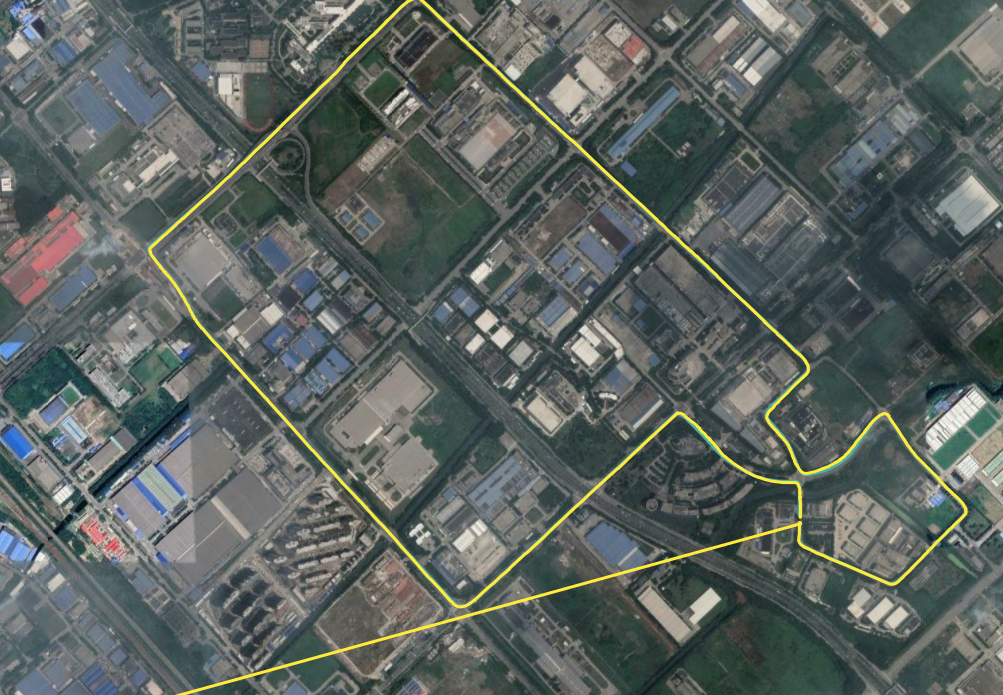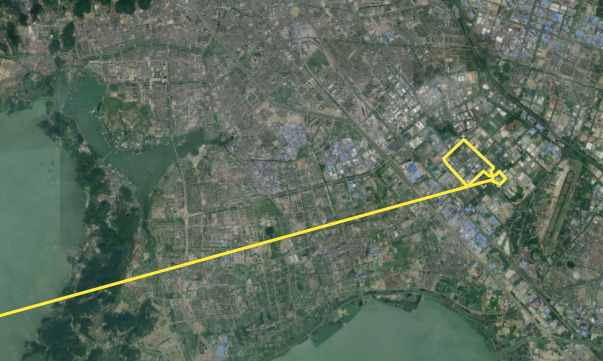For the other four simulations, there is no algorithm trajectory in map_plot, for example, pos_algo_1: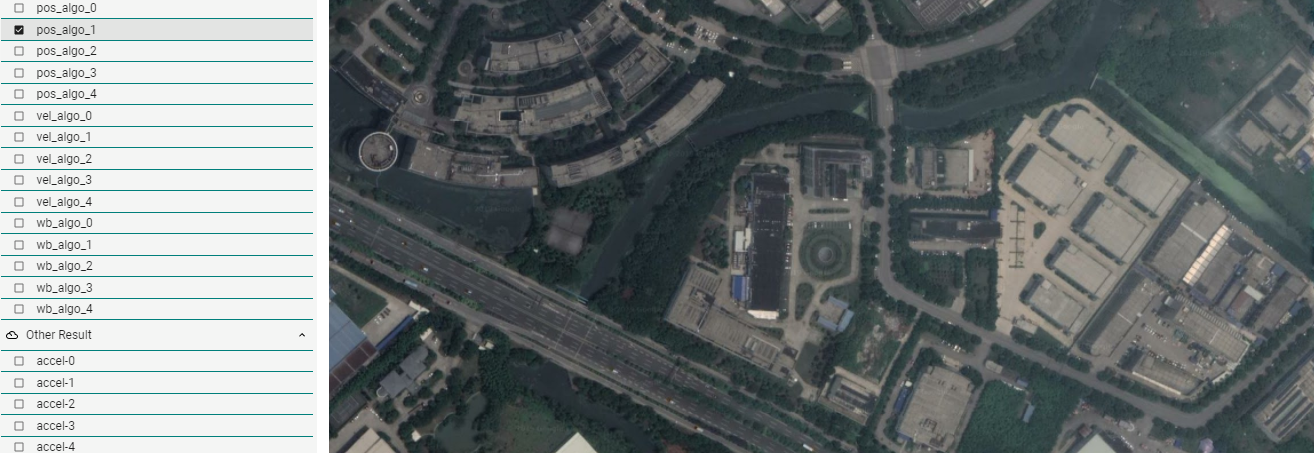(1) ref_pos is generated from a motion profile file. You can consider this an error-free position which is used as a reference. This is not measurement.
(2) If by "very different" you mean the long tail of the trajectory, this is caused by INS solution initialization. Initalizaiton takes some time. Before initialization, zero Lat/Lon/Alt are outputted.
(3) This seems a plot issue. We will fix this. However, for multiple runs of the INS algorithm, because the INS algorithm is written in C and compiled into a shared lib which is called by the simulation written in Python, some reinitialization issue happens when run the shared library multiple times. Please run this algorithm only once. VG is similar. Other demo algorithms are written in Python. You can run them multiple times.

The simulation on Web is only for demo purpose. For serious applications, please use our offline version: https://github.com/Aceinna/gnss-ins-sim. It provides more features and you can get access to all the source code.

@Dong-xiaoguang Thanks, everything is clear now.

@Dong-xiaoguang Sorry to bother you again, as you mentioned, the long tail of trajectory is caused by the INS solution initialization, as a result the error statistics in summary is unreliable. So i download the offline version, but when i try the demo_ins_loose.py in offline version of the simulation, something going wrong.
Here is the screenshot of error message.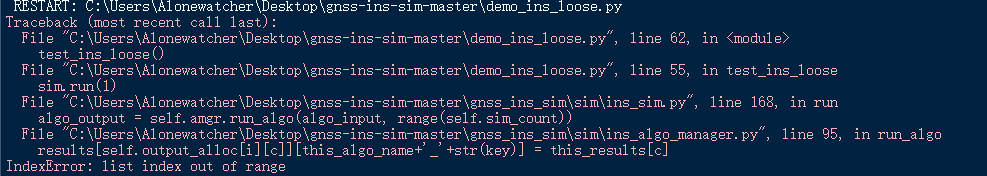I tried to modify the code in ins_algo_manager.py, but failed. Could you give some advice on how to solve this bug?@zhendao-zhao
Sorry for the confusion. demo_ins_loose.py is not complete yet. I planned to make a pure python-based INS algorithm long time ago. But unfortunately, I didn't find enough time to do this. So, ins_loose.py has nothing but a framework. I will add some message to notify users about this.
If you want to try the INS algorithm, please use demo_aceinna-ins.py. If you want further to see the C source code, please refer to
https://developers.aceinna.com/install
The documents and source code are being updated frequently now. Updates are mainly focused on our open source strategies to let user do development on our code base easily. If you have any question, please feel free to ask in the forum.

As I said in the previous reply, the INS algorithm is compiled into a shared library and then called by python code in the simulation. If you want to run it multiple times in one simulation, I can provide a workaround to fix the initialization issue. I only tested the workaround in Win10 x64 with Python 3.7 x64.

aceinna_ins.py

@Dong-xiaoguang Thanks, the workaround works. However, as i mentioned before, when we use the ins algorithm to test long drive demon, the calculation of error_pos_algo includes the data set when the INS algorithm is initialized, and it is clear that just part of the data set leads to wrong position error.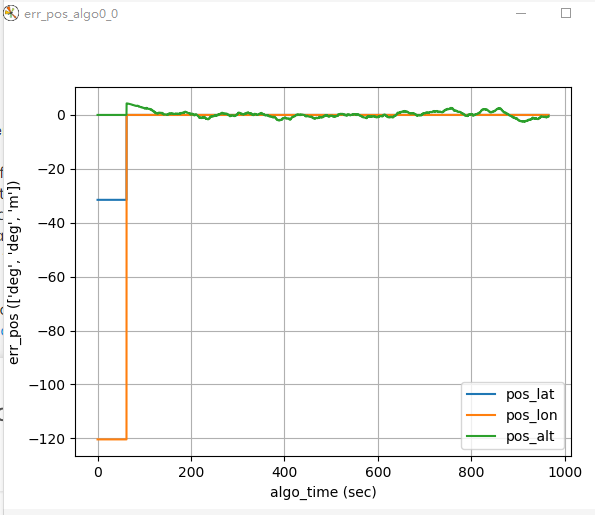As a result, the part of the position error statistics in the summary of the simulation seems unreliable. The max error is the initial latitude and longitude we set in motion file, meanwhile, the Std of error is also unreliable.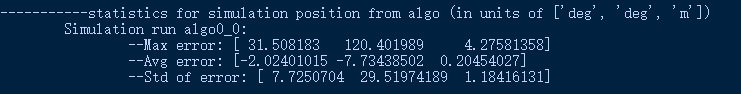I tried to manually delete the useless data generated during the initialization in the pos_algo0_0.csv and ref_pos.csv and recalculate the position error statistics by Excel, but the amount of data between pos_algo0_0.csv and ref_pos.csv is different. For the given demo, one is 24000+, and another is 193000+.
Honestly, i have no idea about how to match the data of the two files.
Could you give me some advice on this problem?

Besides, the unit of the simulation position of ins algorithm is [deg,deg,m], but the free_integration is [m,m,m], in my opinion, the latter is more suitable for analyzing the accuracy of simulation results.
I compared the aceinna_ins.py and free_integration.py in demo_algorithm file to find out how to change the unit of ins result, and found that free_integration import the geoparms from gnss_ins_sim.geoparams, i would like to ask if it is feasible to change the unit by modifying the aceinna_ins.py using related earth parameters.

Thanks a lot for your patience with me.@zhendao-zhao
There are a few things to notice.

1. The algorithm output rate is 25Hz, which is defined in ekfSim_ins.cfg, The max rate can be 100Hz because this algorithm indeed run in an embeded system and there is only limited computational resources. If you want to manually compare the algo output with reference, reference need be downsampled to match the algo output. In the simulation, to deal with cases like this, interp is performaed to align the two sets of data. Please refer to the source code for details.
2. Algo output is in the form of Lat/Lon/Alt. If you want to convert it to [m, m, m], there are two routines in the simulation. But you need to do this manually. The first is to use lla2ecef_batch or lla2ecef in geoparams.py to convert LLA to ECEF positions. However, we usually want position error in NED frame instead of the ECEF frame. So, the second is to further convert the above ECEF position to NED position. You can refer to function array_error in ins_data_manager.py for details.
``````def array_error(self, x, r, angle=False, lla=0):
'''
Calculate the error of an array w.r.t its reference.
Args:
x: input data, numpy array.
r: reference data, same size as x.
angle: True if x contains angles, False if not.
pos: 0 if x is not in LLA form;
1 if x is in LLA form, and NED error is required;
>=2 if x is in LLA form, and ECEF error is required.
Returns:
err: error
'''
if lla == 0:
err = x - r
if angle:
for j in range(len(err.flat)):
err.flat[j] = attitude.angle_range_pi(err.flat[j])
else:
# convert x and r to ECEF first
x_ecef = geoparams.lla2ecef_batch(x)
r_ecef = geoparams.lla2ecef_batch(r)
err = x_ecef - r_ecef
# convert ecef err to NED err
if lla == 1:
n = err.shape
for i in range(0, n):
c_ne = attitude.ecef_to_ned(r[i, 0], r[i, 1])
err[i, :] = c_ne.dot(err[i, :])
return err
``````